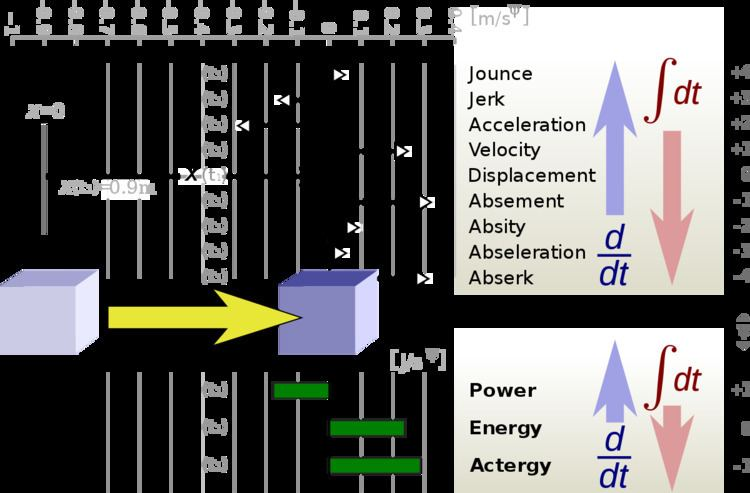# Absement

Updated on
Covid-19
 Common symbols  A SI unit  m·s, m sIn kinematics, absement (or absition) is a measure of sustained displacement of an object from its initial position, i.e. a measure of how far away and for how long. Absement changes as an object remains displaced and stays constant as the object resides at the initial position. It is the first time-integral of the displacement (the area under a displacement vs. time graph), so the displacement is the rate of change (first time-derivative) of the absement. The dimension of absement is length multiplied by time. Its SI unit is meter second (m·s), which corresponds to an object having been displaced by 1 meter for 1 second. This is not to be confused with a meter per second (m/s), a unit of velocity, the time-derivative of position.

## Contents

For example, opening the gate of a gate valve (of rectangular cross section) by 1 mm for 10 seconds yields the same absement of 10 mm·s as opening it by 5 mm for 2 seconds. The amount of water having flown through it is linearly proportional to the absement of the gate, so it is also the same in both cases.

The word absement is a portmanteau of the words absence and displacement. Similarly, absition is a portmanteau of the words absence and position.

## Occurrence in nature

Whenever the rate of change f' of a quantity f is proportional to the displacement of an object, the quantity f is a linear function of the object's absement. For example, when the fuel flow rate is proportional to the position of the throttle lever, then the total amount of fuel consumed is proportional to the lever's absement.

Flow-based musical instruments, such as the hydraulophone, exhibit this phenomenon inherently: whereas a piano produces note strength in proportion to the velocity at which the keys are struck, and the organ (a true tracker organ) produces note strength in proportion to how far down a key is pressed (displacement), the hydraulophone produces note strength in proportion to the time-integral of the distance down the water "key" is pressed. Thus pressing on the key (water jet) for a longer period of time will result in a buildup in sound level, as water accumulates in the sounding mechanism (reservoir), up to a certain maximum filling point beyond which the sound level reaches a maximum, or falls off (along with a slow decay when a water jet is unblocked).

As the displacement can be seen as a mechanical analogue of electric charge, the absement can be seen as a mechanical analogue of the time-integrated charge, a quantity useful for modelling some types of memory elements.

## Applications

In addition to modeling fluid flow and for lagrangian modeling of electric circuits (Jeltsema 2012), absement is used in physical fitness and kinesiology to model muscle bandwidth, and as a new form of physical fitness training. In this context, it gives rise to a new quantity called actergy, which is to energy as energy is to power. Actergy has the same units as action (joule-seconds) but is the time-integral of total energy (time-integral of the Hamiltonian rather than time-integral of the Lagrangian).

Fluid flow in a throttle:

A vehicle's distance travelled results from its throttle's absement. The further the throttle has been opened, and the longer it's been open, the more the vehicle's travelled.

## Relation to PID controllers

PID controllers are controllers that work on a signal that is proportional to a physical quantity (e.g. displacement, proportional to position) and its integral(s) and derivative(s), thusly defining PID in the context of Integrals and Derivatives of a position of a control element in the Bratland sense

depending on the type of sensor inputs, PID controllers can contain gains proportional to position, velocity, acceleration or the time integral of position (absement)…

Example of PID controller (Bratland 2014):

• P, Position;
• I, Absement;
• D, Velocity.
• ## Higher integrals

Just as displacement and its derivatives form kinematics, also displacement and its integrals form "integral kinematics" (Janzen et al. 2014), giving rise to the ordered list of n-th derivatives of displacement (there has been no consensus among physicists on the proper names for derivatives jounce):

## Absement and absementom

Recent work in mechanics and memristors and memcapacitors further builds on the concept of absement, and assigns it the letter "a", and makes extensive use of plots such as the graph of absement as a function of displacement:

…amplitude of the sinusoidal displacement with period

T = 2π/ω,

and a0 = A/ω

is the value about which the analytic absement a(t) oscillates.

See Table 4, "Analytic Displacement and Absement" versus "Piecewise Continuous Displacement and Absement".

## Strain absement

Strain absement is the time-integral of strain, and is used extensively in mechanical systems [Pei etal 2015] and memsprings:

quantity called absement which allows mem-spring models to display hysteretic response in great abundance.

## References

Similar Topics